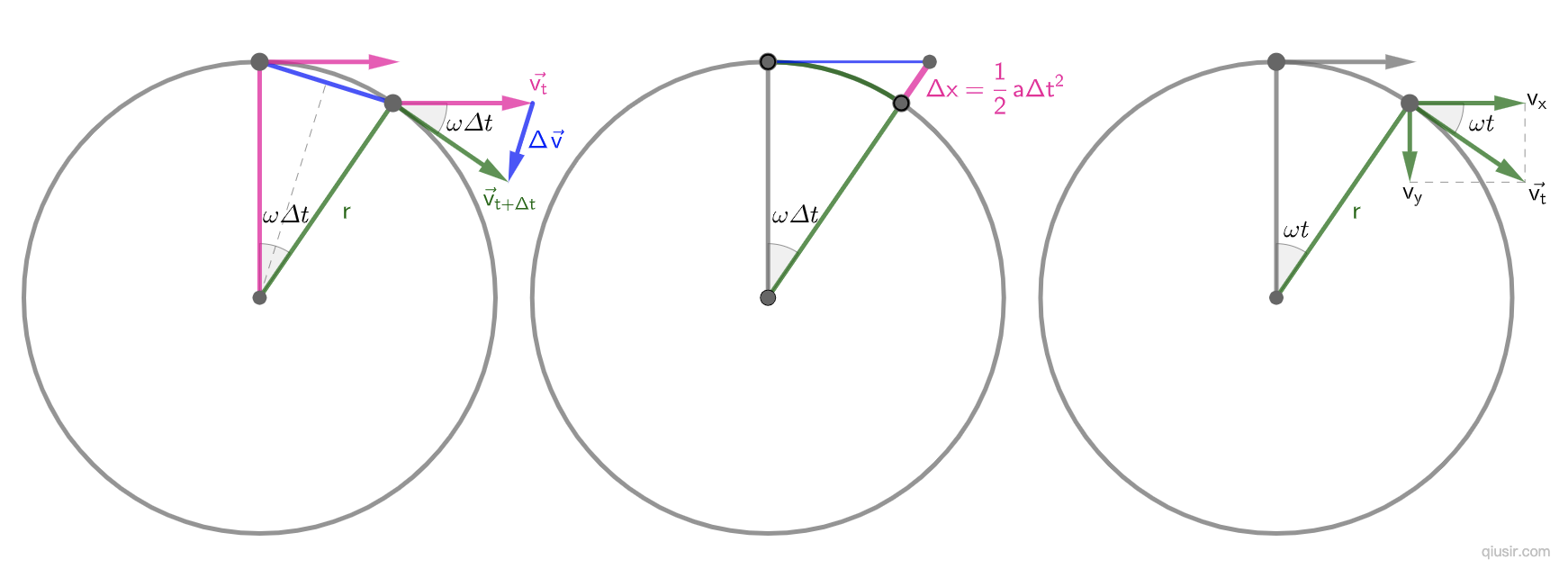06

## 等速圆周的向心力证明$\Delta{v}=\sqrt{2v^2-2v^2cos(\omega\Delta t)}=\sqrt{2}v\sqrt{1-cos(\omega\Delta t)}=\sqrt{2}v\sqrt{2sin^2\frac{\omega\Delta t}{2}}=2vsin\frac{\omega\Delta t}{2}$
$\Delta v=v\omega\Delta t$$\frac{\Delta v}{\Delta t}=v\omega$...

$sin\theta=\frac{y}{r}$$cos\theta=\frac{x}{r}$
$\vec{v}=vsin\theta \hat{x}-vcos\theta \hat{y}=v\frac{y}{r}\hat{x}-v\frac{x}{r}\hat{y}$
$\vec{a}=\frac{d\vec{v}}{dt}$$\vec{a}=\frac{v}{r}(v_y\hat{x}-v_x\hat{y})$
$\left|\vec{a}\right|=\frac{v}{r}\sqrt{v_x^2+v_y^2}=\frac{v^2}{r}$

$\vec{r}(t)=rcos\theta(t)\hat{x}+rsin\theta(t)\hat{y}$
$\vec{v}=\frac{d\vec{r}}{dt}=r(-sin\theta(t))\frac{d\theta}{dt}\hat{x}+rcos\theta(t)\frac{d\theta}{dt}\hat{y}$

$\vec{v}=\omega r(-sin\theta(t))\hat{x}+rcos\theta(t)\hat{y}$
$\vec{a}=\frac{d\vec{v}}{dt}=-\omega^2 r(cos\theta(t))\hat{x}+sin\theta(t)\hat{y}$
$\left|\vec{a}\right|=\omega^2 r$

1937年杨振宁的父亲到西南联大教书，一家人搬到昆明。1938年高二的杨振宁参加大学入学考试（同等学力报考），报考化学系也要求考物理，没有学过高中物理的他借了一本高中物理学在家自学了一个月，觉得物理学很有意思，比化学还有意思，一考入西南联大立刻从化学系转到物理系。

### One Response to “等速圆周的向心力证明”

1. qiusir Says:

余弦近似的一个题目，单摆摆长变为$\frac{1}{9}$，振幅变为$\frac{1}{3}$...